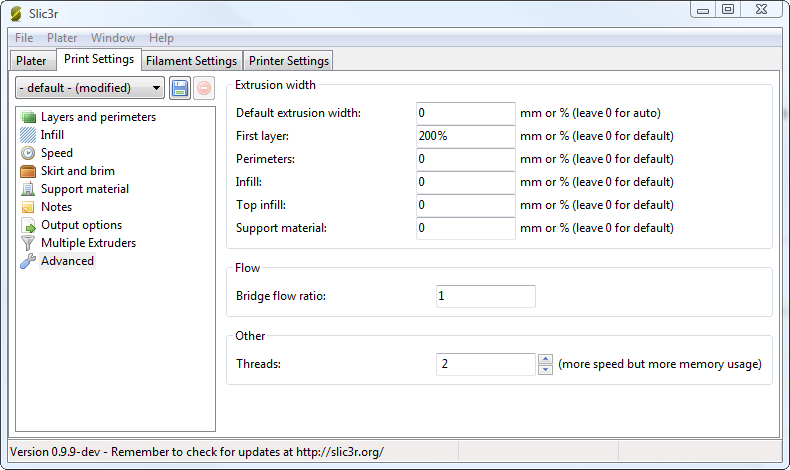# Config for Cetus3d MkII in Slic3r

McErer 2017-12-27 16:04 edit

Hi everybody,

After playing with my new Cetus MkII for a few days I finally managed to find some useful settings for Slic3r.

Tricky parts:

When I started to find the correct settings I first ran into the problem that every g code I wrote tried to move the x axis out of the limits. After removing the additional “G28” (homing commands) everything worked fine. The additional homing seems not to be necessary because the printer has to be initialized after startup anyway.

My Z-offset is 0.2mm above the print bed. To ensure that the nozzle won’t crash the bed I manually wrote some g-code to move the nozzle to all 4 corners and approach the bed slowly to 0.5mm, then I measured the gap between the nozzle and the bed with a feeler gauge. To read the actual value I used the calibration function in the cetus-program.

I printed the first layer of the raft at 350% thickness to close the gap between the nozzle and the bed. The initial idea behind this was to even out a slightly uneven bed.

My settings are far from ideal and just a first step to use this amazing little printer at it’s full potentialHere (https://imgur.com/gallery/96kEe) you can see a comparison between prints using the Cetus slicer and g code generated in Slic3r with my settings.

Greetings,

McErerconfig.zip

Hi,

Thanks for the information. basic question, how do you get file generated by Slic3r to the printer?

In Slic3r you export the g-code (from the "plater" tab), and in Cetus3D you just add the generated file. Simply add it like you would do with a .stl file.

<p>Hi, thank you for sharing this. It works well for me, but I had to change the setting for Extrusion width for the first layer from 200% to 120%.</p><p></p>

Thanks for the slicer info. This machine doesn’t emulate octoprint via wifi, so I am going to have to front-end it with a Pi if I want to run slic3r via the net?

If anyone is interested. This is my final config:

# generated by Slic3r 1.2.9 on Sun May 6 21:52:40 2018 avoid_crossing_perimeters = 0 bed_shape = -180x0,0x0,0x180,-180x180 bed_temperature = 0 before_layer_gcode = bottom_solid_layers = 8 bridge_acceleration = 0 bridge_fan_speed = 100 bridge_flow_ratio = 1 bridge_speed = 60 brim_width = 10 complete_objects = 1 cooling = 1 default_acceleration = 0 disable_fan_first_layers = 3 dont_support_bridges = 1 duplicate_distance = 6 end_gcode = M109 ; switch off extruder\nG0 Z-2;\nG0 X-178 Y2;\nM2 ; end of program external_fill_pattern = rectilinear external_perimeter_extrusion_width = 0 external_perimeter_speed = 50% external_perimeters_first = 0 extra_perimeters = 1 extruder_clearance_height = 20 extruder_clearance_radius = 20 extruder_offset = 0x0 extrusion_axis = E extrusion_multiplier = 24 extrusion_width = 0.48 fan_always_on = 0 fan_below_layer_time = 60 filament_colour = #FFFFFF filament_diameter = 1.75 fill_angle = 45 fill_density = 15% fill_pattern = honeycomb first_layer_acceleration = 0 first_layer_bed_temperature = 0 first_layer_extrusion_width = 0 first_layer_height = 0.2 first_layer_speed = 15 first_layer_temperature = 230 gap_fill_speed = 20 gcode_arcs = 0 gcode_comments = 0 gcode_flavor = reprap infill_acceleration = 0 infill_every_layers = 1 infill_extruder = 1 infill_extrusion_width = 0 infill_first = 0 infill_only_where_needed = 0 infill_overlap = 15% infill_speed = 60 interface_shells = 0 layer_gcode = layer_height = 0.2 max_fan_speed = 100 max_print_speed = 80 max_volumetric_speed = 0 min_fan_speed = 35 min_print_speed = 10 min_skirt_length = 0 notes = nozzle_diameter = 0.4 octoprint_apikey = octoprint_host = only_retract_when_crossing_perimeters = 1 ooze_prevention = 0 output_filename_format = [input_filename_base].gcode overhangs = 1 perimeter_acceleration = 0 perimeter_extruder = 1 perimeter_extrusion_width = 0 perimeter_speed = 35 perimeters = 4 post_process = pressure_advance = 0 raft_layers = 0 resolution = 0 retract_before_travel = 2 retract_layer_change = 0 retract_length = 10 retract_length_toolchange = 10 retract_lift = 0 retract_restart_extra = 0 retract_restart_extra_toolchange = 0 retract_speed = 40 seam_position = aligned skirt_distance = 20 skirt_height = 1 skirts = 1 slowdown_below_layer_time = 5 small_perimeter_speed = 15 solid_infill_below_area = 70 solid_infill_every_layers = 0 solid_infill_extruder = 1 solid_infill_extrusion_width = 0 solid_infill_speed = 20 spiral_vase = 0 standby_temperature_delta = -5 start_gcode = M204 P1500 ; set acceleration\nG1 Z-2;\nG0 X-90 Y90; support_material = 0 support_material_angle = 0 support_material_contact_distance = 0.2 support_material_enforce_layers = 0 support_material_extruder = 1 support_material_extrusion_width = 0 support_material_interface_extruder = 1 support_material_interface_layers = 3 support_material_interface_spacing = 0 support_material_interface_speed = 100% support_material_pattern = pillars support_material_spacing = 4 support_material_speed = 60 support_material_threshold = 0 temperature = 230 thin_walls = 1 threads = 2 toolchange_gcode = top_infill_extrusion_width = 0 top_solid_infill_speed = 15 top_solid_layers = 8 travel_speed = 130 use_firmware_retraction = 0 use_relative_e_distances = 0 use_volumetric_e = 0 vibration_limit = 0 wipe = 0 xy_size_compensation = 0 z_offset = -179.6

I can’t download your config.zip file, seels the link is broken. It cames out this message " Oops! That page doesn’t exist or is private."

config.zip (2.1 KB)
Here you have my current configuration. DO NOT FORGET TO ADJUST THE Z-OFFSET!1 Like